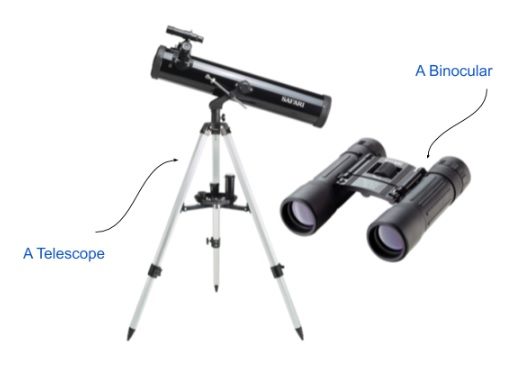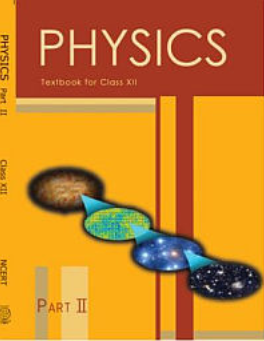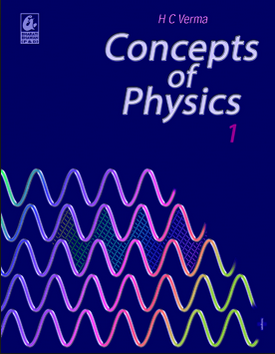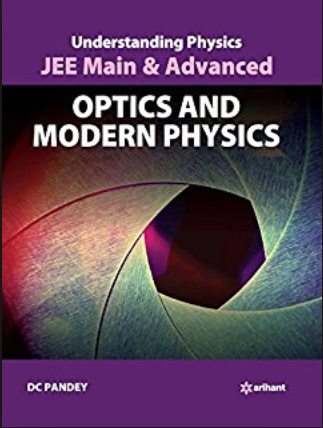# Optics   Share

## What is Optics

Have you ever wondered how the rainbow is formed in the sky? The magic of this eye-pleasing phenomenon lies in the concepts of Optics. Optics is the part of physics which deals with the behaviour of light and how it travels. Without light it was not possible to gain knowledge about the world, imagine a world where there is no light, we could not see anything, then we will have only 4 senses and we would be only able to observe things around us by their touch, smell, the sound they produce and their taste. In our day to day life, we find many applications of optical physics, like the convex mirror is used in cars. The concave mirrors are used in focus lights and telescopes. Similarly, optical lenses are used for correcting eye focus defects like myopia and hypermetropia etc.

Optics is also a very important chapter for various entrance exams including boards. So it is very important to learn the concepts from this chapter to score well. While going through this chapter you will also get to know about various optical devices and their functionality, like Telescope, Binocular and Microscope.## Topics for Optics

General Concepts used in Geometrical Optics, Reflection of Light, Reflection from a Spherical Surface, Refraction of Light and Refractive index of Medium, Law of Refraction, Single Refraction from Plane Surface, Shift due to a Glass Slab, Refraction from Spherical Surface, Lens Theory, Total Internal Reflection, Refraction through Prism, Deviation, Optical Instruments

## Overview of Optics

We discussed that without light we will not be able to see things but do you know that light is just a part of electromagnetic spectra which has wavelengths between 4000and 7000, meaning we can only see a limited amount of electromagnetic radiations. We can’t see X-rays, radio waves, gamma rays etc because their wavelengths are not receptive to human eyes. In this chapter, you will initially study the reflection of light from different types of mirrors like plane mirror, convex and concave mirror. In this chapter, you get introduced to new terminologies so let's understand a few of them which are very basic.

Prepare Online for JEE Main/NEET

Crack JEE 2021 with JEE/NEET Online Preparation Program

1. Centre of curvature - Centre of the sphere of which mirror is a part.

2. Pole - Centre of the mirror surface.

3. Principal Axis - Line which connects Centre of curvature and Pole.

4. Principal Focus - It is the point on the principal axis of a mirror or lens to which parallel rays of light converge or from which they appear to diverge after reflection or refraction.You will then get introduced with the refraction of light through various types of media and shapes, like convex & concave lenses, glass prism and glass slab and liquids etc. Refraction is a phenomenon which occurs when a ray of light travels from one medium to another medium with or without bending. The ray will bend if the refractive indices of both the media will be different from each other and it enters the surface by making an angle with the normal. You must have heard this term ‘refractive index’ but what is the significance of this word?  So basically the speed of light in different media is different and the refractive index is the ratio of the light in two different media. And this difference arises because the wavelength of light changes in the different media. The nature of bending of light depends upon the shape of the media. For example, a convex lens converges the light whereas a concave lens diverges the light.

When you will further proceed in this chapter you will get to know about various optical instruments which use a combination of lenses, most basic optical instruments are simple microscope and telescope.

## Formulas for Optics

• Mirror Formula :• Lateral or linear magnification in Mirror :• Lens Formula :• Lateral or linear magnification in Lens :• Power of a Lens or Mirror (in dioptre) :• Lens maker’s formula :## How to Prepare for Optics

Optics involves a lot of new terminologies and formulas, and there is a very wide range of questions which can be asked from this chapter. It is very important for you to study topic wise and practising questions from the same pattern. It would be easy if you divide this chapter into 3 sections, First reflection from mirrors, Second refraction through the sphere, lenses, slabs and prism and Third Optical instruments. Initially, start with questions which are relevant to a particular section, then after practising a good number of question, try solving questions which involve mix concept. Take mock tests whenever you are confident over a section. Also, try to memorize formulas because many times you will get the direct questions which will involve a formula. Just like other chapters, here also you have to practice a lot to understand Optics better.

## Notes for Optics

• Always keep in mind the sign convention while solving questions for mirror or lens problem.

• Memorize formula after completely understanding the concept, otherwise, you will often get confused as to which formula works for which problem.

• For problems involving multiple optical surfaces always solve unidirectionally, and calculate required values for the previous surface before going to the next surface.

• NCERT book has some very good examples for optics, please read them carefully.

• General Tip: While practising questions always write what quantities are given in the question and what you have to find.

## Recommended books for Optics

You can start with NCERT for theory as well as for questions, this will surely help you to build your concepts. After completing NCERT book, solve questions from NCERT Exemplar book and for more advanced level questions you could either follow Concepts of Physics by H.C. Verma or Understanding  Physics by DC Pandey.

## Physics Chapter wise Notes For Engineering and Medical Exams

 Chapters No. Chapters Name Chapter 1 Physics and Measurement Chapter 2 Kinematics Chapter 3 Laws of motion Chapter 4 Work Energy and Power Chapter 5 Rotational Motion Chapter 6 Gravitation Chapter 7 Properties of Solids and Liquids Chapter 8 Kinetic theory of Gases Chapter 9 Thermodynamics Chapter 10 Oscillations and Waves Chapter 11 Electrostatics Chapter 12 Current Electricity Chapter 13 Magnetic Effects of Current and Magnetism Chapter 14 Electromagnetic Induction and Alternating currents Chapter 15 Electromagnetic Waves Chapter 17 Dual Nature of Matter and Radiation Chapter  18 Atoms And Nuclei Chapter 19 Electronic devices Chapter 20 Communication Systems Chapter 21 Experimental skills

### Topics from Optics

• Reflection and refraction of light at plane and spherical surfaces ( JEE Main ) (23 concepts)
• mirror formula ( JEE Main ) (9 concepts)
• Deviation and Dispersion of light by a prism, ( JEE Main ) (7 concepts)
• Lens Formula, Magnification ( JEE Main ) (2 concepts)
• Power of a Lens, Combination of thin lenses in contact ( JEE Main ) (8 concepts)
• Resolving power of microscopes and astronomical telescopes ( JEE Main ) (4 concepts)
• Microscope and Astronomical Telescope (reflecting and refracting) and their magnifying powers. ( JEE Main ) (2 concepts)
• Expression for fringe width,coherent sources and sustained interference of light ( JEE Main ) (10 concepts)
• Interference,Young's double slit experiment ( JEE Main ) (6 concepts)
• Diffraction due to single slit, width of central maximum ( JEE Main ) (3 concepts)
• Polaristaion, Plane polarized light ( JEE Main ) (1 concepts)
• Reflection of light ( JEE Main ) (11 concepts)
• Refraction of light ( JEE Main ) (5 concepts)
• Refraction through a Prism ( JEE Main ) (4 concepts)
• Lenses ( JEE Main ) (12 concepts)
• Optical Instruments ( JEE Main ) (5 concepts)
• Introduction to wave optics ( JEE Main ) (4 concepts)
• Polarization of light ( JEE Main ) (3 concepts)
• Interference and Diffraction of light wave ( JEE Main ) (11 concepts)
• Resolving power of optical instruments ( JEE Main ) (3 concepts)

### Important Books for Optics

•••Exams
Articles
Questions